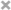# Xiuchuan Zhang

Personal Website

This is Xiuchuan's personal website.
I plan to post some of my current learning and review notes on it.
If you have any questions or suggestions, welcome to comment in my posts.

These are the notes by learning the “Introduction to Probability and Data” from Coursera.org for future reviews.

## Introduction to Data

• Data matrix: data are organized in
• Observation (case): row
• Variable: column

### Types of variables

• Numerical
• numerical values (sensible to add, subtract, take averages, etc. with them) 1. continuous: infinite number of values within a given range 2. discrete: specific set of numeric values
• Categorical
• limited number of distinct categories (not sensible to do arithmetic operations)
1. ordinal: inherent ordering
2. Nominal: not ordering

### Relationships between variables

• associated (dependent) : positive or negative
• independent : not associated

### Observational study

• collect data in a way that does not directly interfere with how data arise (“observe”)
• only establish an association
• retrospective: use past data
• prospective: data are collected throughout the study

### Experiment study

• randomly assign subjects to treatments
• establish causal connections

### Why not Census

• some individuals are hard to locate or measure, and these people be different from the rest of the population
• populations rarely stand still

### Sources of Sampling bias

• Convenience sample: individuals who are easily accessible are more likely to be included in the sample
• Non-response: If only a (non-random) fraction of the randomly sampled people respond to survey such that the sample is no longer representative of the population
• Voluntary response: Occurs when the sample consists of people who volunteer to respond because they have strong opinions on the issue

### Sample Methods

• simple random sampling: randomly select cases from the population
• stratified sampling: first divide the population into homogenous groups called strata, and then randomly sample from within each stratum
• cluster sampling: divide the population into clusters, randomly sample a few clusters, and then sample all observation within these clusters
• multistage sampling: divide the population into clusters, randomly sample a few clusters, and then we randomly sample observations from within these clusters

### Experimental design

• Principles of Experimental Design:
1. control: compare treatment of interest to a control group
2. randomize: randomly assigning subjects to treatments
3. replicate: collect a sufficiently large sample, or replicate the entire study
4. block: block for variables known or suspected to affect the outcome
• confounding variable: is correlated with both the explanatory and response variables
• Explanatory variables (factors): conditions we can impose on our experimental units
• Blocking variables: characteristics that the experimental units come with, that we would like to control for
• Blocking is like stratifying:
• blocking during random assignment
• stratifying during random sampling

### Experimental terminology

• placebo: fake treatment, often used as the control group for medical studies
• placebo effect: showing change despite being on the placebo
• blinding: experimental units do not know which group they are in
• double-blind: both the experimental units and the researchers do not know the group assignment

### Random sampling and random assignment

ideal experiment $\searrow$ Random Assignment No Random Assignment most observational studies $\swarrow$
Random Sampling Causal and Generalizable not Causal, but Generalizable Generalizability
No Random Sampling Causal, but not Generalizable neither Causal nor Generalizable Np Generalizability
most experiments $\nearrow$ Causation Association bad observational studies $\nwarrow$

## Exploratory Data Analysis and Introduction to Inference

### Scatterplots

• explanatory variable on x axis
• response variable on y axis
• correlation, not causation

### Evaluate the relationship

• direction: positive or negative
• shape: linear or curved or others
• strength: strong or weak
• outliers

### Histogram

• provide a view of the data density
• especially useful for identifying shapes of distributions

### Skewness

• distributions are skewed to the side of the long tail
• left skewed: the longer tail is on the left on the negative end
• mean < median
• symmetric: no skewness is apparent
• mean $\approx$ median
• right skewed: the longer tail is on the right, the positive end
• mean > median

### Modality

• unimodal: one prominent peak (normal distribution or bell curve)
• bimodal: two prominent peak (might two distinct groups in data)
• uniform: no prominent peaks (no apparent trend)
• multimodal: more than two prominent peaks

### Bin width

the chosen bin width can alter the story the histogram is telling

• bin width too wide: might lose interesting details
• bin width too narrow: might be difficult to get an overall picture of the distribution
• ideal bin width depends on the data you are working with

### Dot plot

• useful when individual values are of interest
• can get too busy as the sample size increases

### Box plot

• useful for highlighting outliers, media, IQR(interquartile range)

### Intensity map

• useful for highlighting the spatial distribution

• range: (max - min)
• variance: roughly the average squared deviation from the mean
• sample variance: $s^2$
• population variance: $(\sigma)^2$
• $s^2 = \frac{\sum_{i=1}^{n} (x_i - \bar{x})^2}{n-1}$
• standard deviation: roughly the average deviation around the mean, and has the same units as the data
• sample sd: $s$
• population sd: $\sigma$
• inter-quartile range
• range of the middle 50% of the data, distance between the first quartile (25th percentile) and third quartile (the 75th percentile)
• most readily available in a box plot.
• $IQR = Q_3 - Q_1$

### Robust Statistics

• define: measures on which extreme observations have little effect
• robust measures of center & spread:
robust non-robust
center median mean

### Transforming data

• define: a rescaling of the data using a function
• When data are very strongly skewed, we sometimes transform them, so that they are easier to model
• (natural) log transformation:
• often applied when much of the data cluster near zero (relative to larger values in the dataset) and all observations are positive
• to make the relationship between the variables more linear, and hence easier to model with simple methods
• other transformations:
• square root
• inverse
• goals:
• to see the data structure differently
• to reduce skew assist in modeling
• to straighten a nonlinear relationship in a scatterplot

### Exploring Categorical Variables

• Bar plots
• Q: How are bar plots different than histograms?
• barplots for categorical variables, histograms for numerical variables
• x-axis on a histogram is a number line, and the ordering od the bars are not interchangeable
• Segmented bar plot
• useful for visualizing conditional frequency distributions
• compare relative frequencies to explore the relationship between the variables
• Relative frequency segmented bar plot
• Mosaicplot
• Side-by-side box plots

### Introduction to inference

• null hypothesis($H_0$): independent, “There is nothing going on”
• alternative hypothesis($H_A$): dependent, “There is something going on”

• hypothesis testing framework
• start with a null hypothesis($H_0$) that represents that status quo
• set an alternative hypothesis($H_A$) that represents our research question, i.e. what we’re testing for
• conduct a hypothesis test under the assumption that the null hypothesis is true, either via simulation or using theoretical methods
• If the test results suggest that the data do not provide convincing evidence for the alternative hypothesis, we stick with the null hypothesis
• If they do, then we reject the null hypothesis in favor of the alternative

### Inference summary

• set a null and an alternative hypothesis
• simulate the experiment assuming that the null hypothesis is true
• evaluated the p_value: probability of observing an outcome at least as extreme as the one observed in the original data
• if this probability is low, reject the null hypothesis in favor of the alternative

## Probability and Distribution

• random process: know what outcomes could happen, but don’t know which particular outcome will happen

• P (A) = Probability of event A
• 0≤P(A)≤1
• frequentist interpretation: The probability of an outcome would occur if we observed the random process an infinite number of times.

• Bayesian interpretation: A Bayesian interprets probability as a subjective degree of belief

• largely popularized by revolutionary advance in computational technology and methods during the last twenty years

• law of large members: sates that as more observations are collected, the proportion of occurrences with a particular outcome converges to the probably of that outcome

• common misunderstanding: gambler’s fallacy (law of averages)

• disjoint (mortally exclusive) events cannot happen at the same time
• P(A & B) = 0
• Union of disjoint events: P(A or B) = P(A) + P(B) - P(A & B)
• Complementary → disjoint; complementary !← disjoint
• non-disjoint events can happen at the same time
• P(A & B) != 0
• sample space: a collection of all possible outcomes of a trial

• probability distribution: all possibility outcomes in the sample space, and the probabilities with they occur

• Rules:
• the events listed must be disjoint
• each probability must be between 0 and I
• the probabilities must total I
• complementary events: two mentally exclusive events whose probabilities add up to l
• Independence: P(A/B) = P(A), P(A1, … & Ak<\sub>) = P(A1) × … × P(Ak)

• Dependence: P(A/B) = P(A & B)/ P(B), P(A & B) = P(A/B) × P(B)

• Posterior Probability: P(hypothesis / data) → P(hypothesis is true / observed data)

• P-value: P(data / hypothesis) → P(observed or more extreme outcome / H0 is true)

## Normal Distribution

### Normal distribution $N( \mu , \sigma )$

• unimodal and symmetric
• bell curve
• follows very strict guidelines about how variably the data are distributed around the mean
• Many variables are nearly normal, but none are exactly normal
• two parameters: mean μ and stand deviation σ
• Changing the center and the spread of the distribution changes the overall shape of the distribution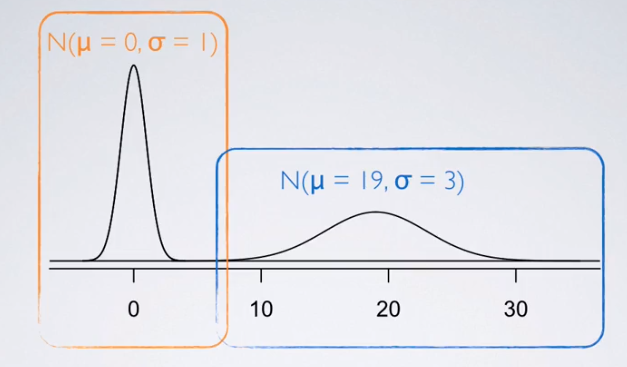• rules govern the variability of normally distributed data around the mean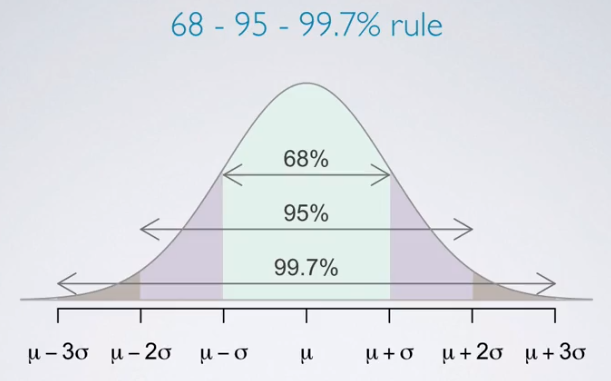### Standardizing with Z scores

• standardized (Z) score of an obervation is the number of standard deviations it falls above or below the mean
• $Z = \frac{observation - mean}{SD}$
• Z score of mean = 0 (normally: median ≈ 0 )
• unusual observation: $\lvert Z\rvert > 2$
• defined for distributions of any shape
• when the distribution is normal, Z scores can be used to calculate percentiles
• Percentile is the percentage of observations that fall below a given data point
• graphically, percentile is the area below the probability distribution curve to the left of that observation
• if the distribution does not follow the nice unimodal symmetric normal shape, you’d need to use calculus for that
• Methods for Z scores
1. Using R: pnorm(-1, mean = 0, sd = 1) (qnorm for quantiles or cutoff values)
2. Distribution Calculator
3. Table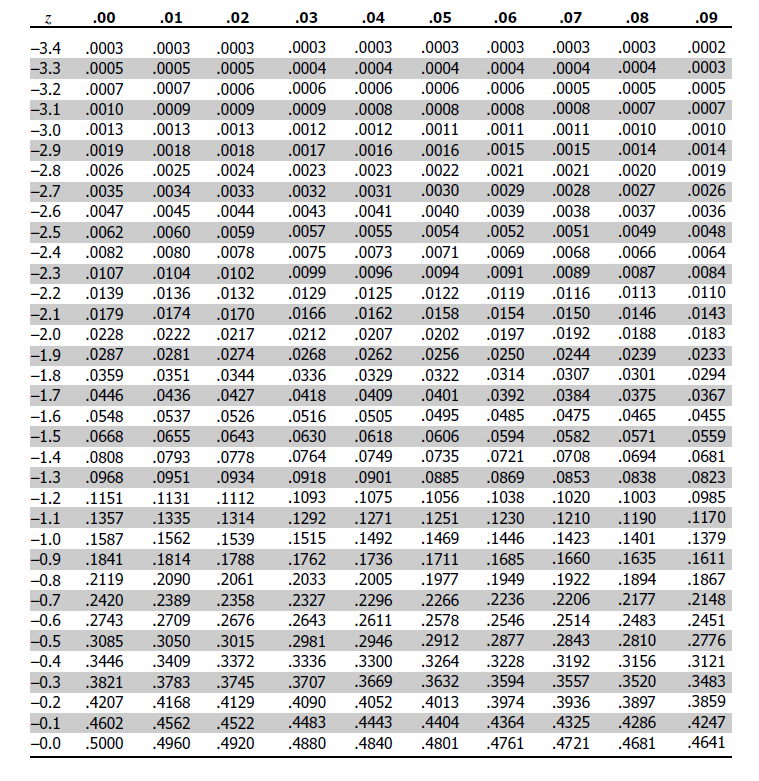### Evaluating

• anatomy of a normal probability plot
• Data are plotted on the y-axis of a normal probability plot, and theoretical quantiles (following a normal distribution) on the x-axis
• If there is a one-to-one relationship between the data and the theoretical quantiles, then the data follow a nearly normal distribution.
• Since a one-to-one relationship would appear as a straight line on a scatter plot, the closer the points are to a perfect straight line, the more confident we can be that the data follow a normal model.
• Constructing a normal probability plot requires calculating percentiles and corresponding z-scores for each observation, which is tedious. Therefore, we generally rely on software when making these plots.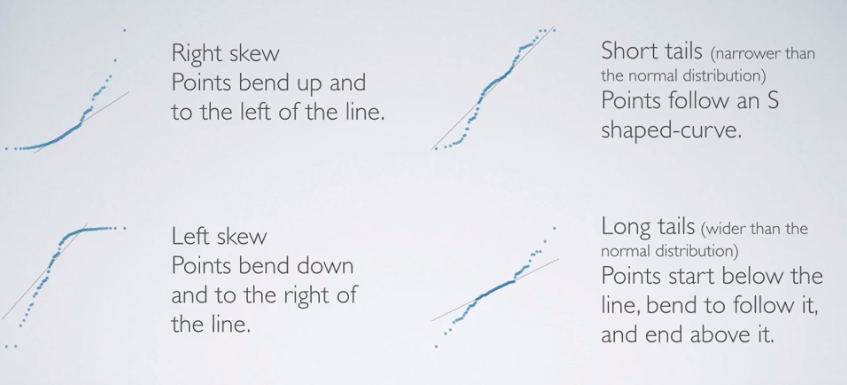• Also can using 68-95-99.7% rule

## Binomial Distribution

• binomial distribution describes the probability of having exactly k successes in n independent Bernoulli trials with probability of success p
• # of scenarios × P(single scenario)
• $P(k = K) = {n \choose k} p^k (1-p)^{(n-k)}$

in R: dbinom(k, size, p) Distribution Calculator

• Choose function: ${n \choose k}=\dfrac{n!}{k!(n−k)!}$

in R: choose(n, k)

### Binomial conditions

1. The trials are independent.
2. The number of trials, n, is fixed.
3. Each trial outcome can be classified as a success or failure.
4. The probability of a success, p, is the same for each trial.
• Expected value (mean) of binomial distribution ($\mu = np$) and its standard deviation ($\sigma = \sqrt{np(1-p)}$)

### normal approximation

• Fact: when the number of trials increases, the shape of the binomial actually starts looking closer and closer to a full normal distribution

• Calculate the probabilities for each outcome from a to b and sum them up

in R: sum(dbinom(a:b, size = n, p =p))

• Success-failure rule: a binomial distribution with at least 10 expected successes and 10 expected failures closely follows a normal distribution
• $np \geq 10$
• $n( 1-p ) \geq 10$
• Normal approximation to the binomial: If the success-failure condition holds, then
• $Binomial(n,p) \thicksim Normal(\mu,\sigma)$
• where $\mu = np$ and $\sigma = \sqrt{np(1-p)}$

Support